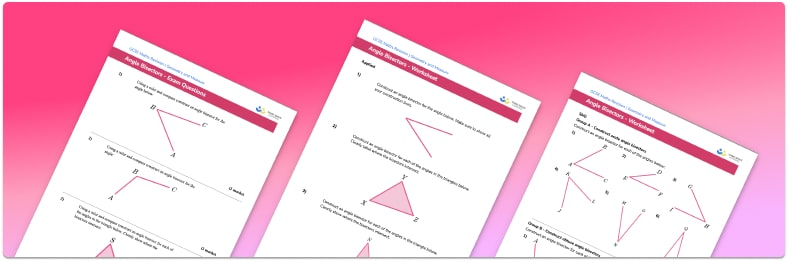# Angle bisector worksheet• Section 1 of the angle bisector practice worksheet contains 21 skills-based angle bisector questions, in 3 groups to support differentiation
• Section 2 contains 3 applied angle bisector questions with a mix of worded problems and deeper problem solving questions
• Section 3 contains 3 foundation and higher level GCSE exam style angle bisector questions
• Answers and a mark scheme for all angle bisector questions are provided
• Questions follow variation theory with plenty of opportunities for students to work independently at their own level
• All questions and resource types created by fully qualified expert secondary maths teachers
• Suitable for GCSE maths revision for AQA, OCR and Edexcel exam board

• This field is for validation purposes and should be left unchanged.

You can unsubscribe at any time (each email we send will contain an easy way to unsubscribe). To find out more about how we use your data, see our privacy policy.

### Angle bisector at a glance

The angle bisector of a given angle is a line drawn which cuts the angle in half (bisects it). To bisect an angle you will need a pencil, a pair of compasses and a straight edge (ruler). Although we are working with angles, we do not use a protractor when bisecting an angle.

In order to bisect the angle ABC, start by placing the point of the compasses on the vertex of the angle, at B. Draw an arc which intersects both lines AB and BC. Next move the point of the compasses to one of these intersections and draw another arc in the middle of the angle. Repeat this step with the point of the compasses on the other intersection. Finally, draw a straight line from the vertex of the angle to the intersection of the two middle arcs.

The angle bisector theorem states that an angle bisector of an angle in a triangle divides the opposite side in two segments that are proportional to the other two sides of the triangle.

Looking forward, students can then progress to additional geometry worksheets, for example a congruent triangles worksheetFor more teaching and learning support on Geometry our GCSE maths lessons provide step by step support for all GCSE maths concepts.

## Do you have KS4 students who need more focused attention to succeed at GCSE?There will be students in your class who require individual attention to help them succeed in their maths GCSEs. In a class of 30, it’s not always easy to provide.

Help your students feel confident with exam-style questions and the strategies they’ll need to answer them correctly with our dedicated GCSE maths revision programme.

Lessons are selected to provide support where each student needs it most, and specially-trained GCSE maths tutors adapt the pitch and pace of each lesson. This ensures a personalised revision programme that raises grades and boosts confidence.

Find out more